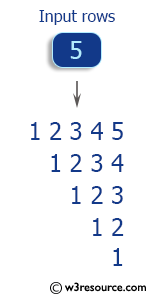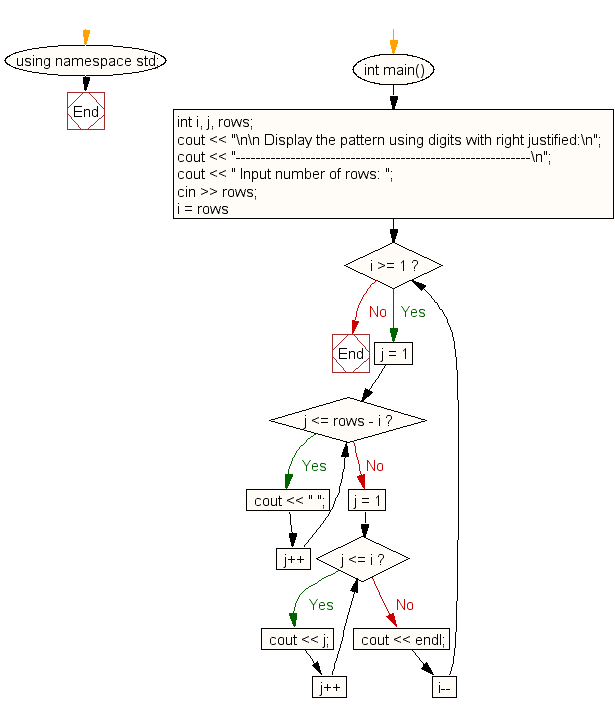﻿ C++ : Display the pattern like highest columns in first row

# C++ Exercises: Display the pattern using digits with right justified and the highest columns appears in first row

## C++ For Loop: Exercise-51 with Solution

Write a C++ program that displays the pattern with the highest columns in the first row and digits with the right justified digits.

Pictorial Presentation:Sample Solution:

C++ Code :

``````#include <iostream>
using namespace std;

int main()
{
int i, j, rows;
cout << "\n\n Display the pattern using digits with right justified:\n";
cout << "-----------------------------------------------------------\n";
cout << " Input number of rows: ";
cin >> rows;
for (i = rows; i >= 1; i--)
{
for (j = 1; j <= rows - i; j++)
cout << " ";
for (j = 1; j <= i; j++)
cout << j;
cout << endl;
}
}
``````

Sample Output:

``` Display the pattern using digits with right justified:
-----------------------------------------------------------
Input number of rows: 5
12345
1234
123
12
1
```

Flowchart:C++ Code Editor: